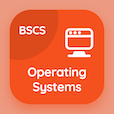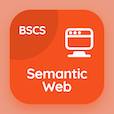Computer Science Courses Online

Digital Logic Design Practice Tests

Digital Logic Design Online Tests

The Book Introduction to Algorithmic State Machine Multiple Choice Questions (MCQ Quiz) with answers, Introduction to Algorithmic State Machine MCQ Quiz PDF download to study online logic design degree courses. Practice Algorithmic State Machine Multiple Choice Questions and Answers (MCQs), Introduction to Algorithmic State Machine quiz answers PDF for computer science programs. The e-Book Introduction to Algorithmic State Machine MCQ App Download: flip flop, timing in state machines, registers in dld, asm chart test prep for computer software engineer.

The MCQ: How many selected lines does an 8-line to 1-line multiplexer require? PDF, "Introduction to Algorithmic State Machine" App Download (Free) with 2, 3, 4, and 8 choices for computer science programs. Study introduction to algorithmic state machine quiz questions, download Google eBook (Free Sample) for cheapest online computer science degree.

## Logic Design MCQs: Introduction to Algorithmic State Machine Quiz Questions

MCQ: How many selected lines does an 8-line to 1-line multiplexer require?

A) 2
B) 3
C) 4
D) 8

MCQ: A sequential circuit is also called

A) state
B) encoder
C) flip-flop
D) state machine

MCQ: ASM stands for

A) Algorithmic State Machine
B) Algorithmic Solid Machine
C) Arithmetic State Machine
D) Arithmetic Solid Machine

MCQ: A method used to specify the sequence of the algorithm is

A) map
B) data
C) flowchart
D) operation

### Practice Tests: Digital Logic Design Exam Prep

Download Digital Logic Design Quiz App, Operating Systems MCQ App, and Semantic Web MCQs App to install for Android & iOS devices. These Apps include complete analytics of real time attempts with interactive assessments. Download Play Store & App Store Apps & Enjoy 100% functionality with subscriptions!